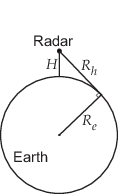# horizonrange

## Syntax

```Rh = horizonrange(H) Rh = horizonrange(H,Re) Rh = horizonrange(___,'SurfaceHeight',surfht) ```

## Description

`Rh = horizonrange(H)` returns the horizon range, `Rh`, of a radar system `H` meters above the surface. The computation uses an effective earth radius of approximately 4/3 times the actual earth radius.

`Rh = horizonrange(H,Re)` specifies the effective earth radius, `Re`.

`Rh = horizonrange(___,'SurfaceHeight',surfht)` also specifies the surface height, `surfht`.

## Input Arguments

 `H` Height of radar system above surface, specified as a scalar or a length-M vector. Units are in meters. `Re` Effective earth radius, specified as a positive scalar. Units are in meters Default: `effearthradius`, which is approximately 4/3 times the actual earth radius
 `surfht` Height of earth surface at the horizon, specified as a scalar or length-M vector. This input can also be interpreted as the height of significant ground clutter at the horizon. If `H` and `surfht` are vectors, their lengths must be equal. Defaults to 0 m.

## Output Arguments

 `Rh` Horizon range in meters of radar system at altitude `H`.

## Examples

collapse all

Find the range to the horizon from an antenna that is 30 m high.

`R = horizonrange(30)`

collapse all

### Horizon Range

The horizon range of a radar system is the distance from the radar system to the earth along a tangent. Beyond the horizon range, the radar system detects no return from the surface through a direct path.The value of the horizon range is:

`$\sqrt{2{R}_{e}H+{H}^{2}}$`

where Re is the effective earth radius and H is the altitude of the radar system.

## References

 Long, Maurice W. Radar Reflectivity of Land and Sea, 3rd Ed. Boston: Artech House, 2001.

 Skolnik, M. Introduction to Radar Systems, 3rd Ed. New York: McGraw-Hill, 2001.

## Extended Capabilities